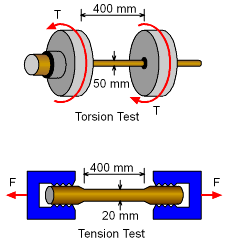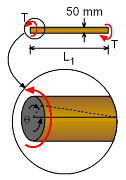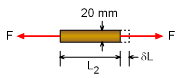Ch 2. Torsion Multimedia Engineering Mechanics CircularBars Nonuniform &Indeterminate Thin-walledTubes Non-Circular Bars
 Chapter 1. Stress/Strain 2. Torsion 3. Beam Shr/Moment 4. Beam Stresses 5. Beam Deflections 6. Beam-Advanced 7. Stress Analysis 8. Strain Analysis 9. Columns Appendix Basic Math Units Basic Equations Sections Material Properties Structural Shapes Beam Equations Search eBooks Dynamics Fluids Math Mechanics Statics Thermodynamics Author(s): Kurt Gramoll ©Kurt GramollMECHANICS - EXAMPLE ExampleTest Specimens To determine mechanical properties of a material, a torsion test and a tension test was done for two different diameter bar made from the same material as shown in the figures on the left. During the torsion test, a maximum shear stress of 100 MPa was measured on a bar 50 mm diameter. The angle of twist was 2.5 degree over a length of 400 mm. The tensile test was done with a bar of 20 mm diameter and 400 mm length. The specimen had an elongation of 0.5 mm when subjected to a tensile load of 40 kN. Determine the Poisson's ratio of the material. Solution Torsion test data:      Diameter of bar, d1 = 2r1 = 50 mm      Length, L1 = 400 mm      Maximum shear stress, τ = 100 MPa      θ = 2.5° = 0.04363 rad Tension test data:      Diameter of bar, d2 = 2r2 = 20 mm      Length, L2 = 400 mm      Tension, F= 40 kN      Elongation, δL= 0.5 mm To determine the poisson's ratio, it is necessary to know the modulus of elasticity and shear modulus of the material. These can be determined using the data from the two tests.Torsion Test Details For the tension test      Strain,      ε = Elongation / Length        = δL / L2        = 0.5 / 400        = 0.00125      Stress,      σ = F / A         = F / (π r22)         = 40 / (π 0.012)         = 127,300 kPa            = 127.3 MPa      Modulus of Elasticity,      E = σ / ε         = 127.3 / 0.00125         = 101,860 MPa         = 101.9 GPaTension Test Details For the torsion test      Shear Modulus,      G = L1 τ / θ r1         = (400)(100) / (0.04363)(50/2)         = 36,672 MPa         = 36.67 GPa Now for Poisson's ratio,      G = E / 2(1 + ν)      ν = (E / 2G) - 1        = {101.9 / (2)(36.67)} - 1        = 0.3894 Poisson's ratio, ν = 0.389

Practice Homework and Test problems now available in the 'Eng Mechanics' mobile app
Includes over 400 problems with complete detailed solutions.
Available now at the Google Play Store and Apple App Store.